# PSAT Math Practice: Calculator

This practice test covers the second section of PSAT Math. For these questions the use of a calculator is allowed.

Congratulations - you have completed .

You scored %%SCORE%% out of %%TOTAL%%.

Your performance has been rated as %%RATING%%

 Question 1

### How much money does Joe spend at this restaurant in each month that has 31 days?

 A $\$837.84$B$\$928.07$ C $\$153.45$D$\$920.70$
Question 1 Explanation:
The correct answer is (D). The cost of one night of food is:

$\$24.75 + (0.08)(24.75) + (0.12)(24.75) = \$29.70$

Multiply by 31 to find his monthly expenditure:

$\$29.70 × 31 = \$920.70$
 Question 2

### The scatterplot below shows the number of households with 3 cars from 2000 to 2010 in a small community.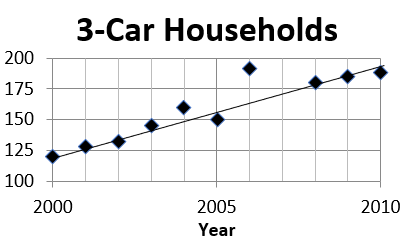### Based on the line of best fit shown, which of the following values is closest to the average yearly increase in the number of households with 3 cars?

 A $5$ B $7$ C $11$ D $15$
Question 2 Explanation:
The correct answer is (B). Use the scatterplot to estimate these values:

2001 = 125 households

2008 = 175 households

$\text{Average}$ $= \dfrac{175 − 125}{7}$ $= \dfrac{50}{7} ≅ 7$
 Question 3

### If $k$ is a positive constant not equal to 1, which of the following could be a graph of $y + x = k(x − y)$ in the $xy$-plane?

 A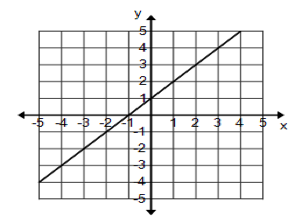BC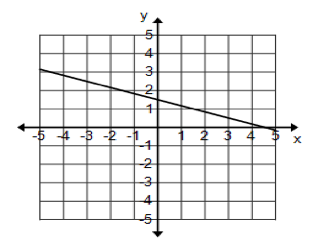D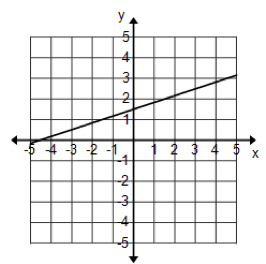Question 3 Explanation:

$y + x = k(x − y)$

$y + x = kx − ky$

$y + ky = kx – x$

$y(1 + k) = x(k − 1)$

$y = \dfrac{k − 1}{k + 1}x$

So, when $x = 0$, $y = 0$. This means the line has to pass through the origin. Only answer choice (B) shows a line through the origin.
 Question 4

### The height of an airplane is increasing at a constant rate. Ten minutes after takeoff, the plane is 1,225 feet above sea level. Twenty-five minutes after takeoff, the plane is 15,570 feet above sea level. Which of the following is the value of the constant rate at which the plane’s height is increasing per minute?

 A $956.33$ B $965.33$ C $8338.33$ D $14,345.00$
Question 4 Explanation:
The correct answer is (A). The two points of the plane are (10, 1,225) and (25, 15,570). The rate at which the height is increasing per minute is:

$\dfrac{15570 - 1225}{25 - 10}$ $= 956.33 \text{ feet/minute}$
 Question 5

### One Gigabyte is 1024 Megabytes. A DVD can hold 4.7 Gigabytes of data. A floppy disc can hold 1.44 Megabytes of data. What is the maximum number of full floppy disks whose data will fit into one DVD?

 A $3342$ B $3343$ C $3555$ D $3558$
Question 5 Explanation:
The correct answer is (A). One DVD can hold:

$\require{cancel} 4.7 \cancel{\text{ GB}} \cdot \dfrac{1024 \text{ MB}}{1 \cancel{\text{ GB}}}$ $= 4812.8 \text{ MB}$

$\require{cancel} 4812.8 \cancel{\text{ MB}} \cdot \dfrac{1 \text{ Full Disk}}{1.44 \cancel{\text{ MB}}}$ $= 3342.222 \text{ Full Disks}$

Since we are considering only full disks, the largest whole number less than $3342.222$ is $3342$.
 Question 6

### In a historical town of Alexandria, the number of voters for each of four different age groups is shown for three different years.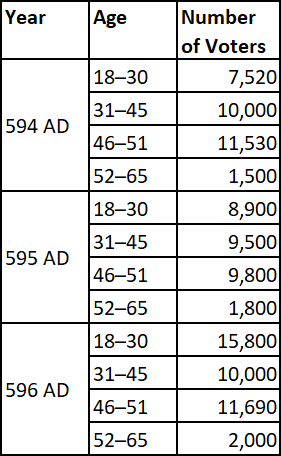A $18–30$ B $31–45$ C $46–51$ D $52–65$
Question 6 Explanation:
The correct answer is (A). The number of voters from 18 to 30 increased by:

$15{,}800 − 7{,}520$ $= 8{,}280 \text{ voters}$

This is a much greater increase than that of the other three age groups.
 Question 7### According to the table, approximately what percent of voters were 46–65 years old in 596 AD?

 A $35\%$ B $45\%$ C $50\%$ D $55\%$
Question 7 Explanation:
The correct answer is (A). To find the percent of voters 46–65 years old in 596 AD:

$\text{Percent} = \frac{\text{Voters 46–65}}{\text{Total Voters}}$

$= \frac{11,690 + 2,000}{15,800 + 10,000 + 11,690 + 2,000}$

$= \frac{13,690}{39,490} ≃ 0.35 = 35\%$
 Question 8

### The graphs of a system of equations—a parabola, a straight line, and a circle—are shown in the figure below.### How many solutions does the system have?

 A $0$ B $1$ C $2$ D $3$
Question 8 Explanation:
The correct answer is (C). Solutions to the system are points where all three equations intersect. This occurs at two coordinates only.
 Question 9
$x^2 + y^2 = 185$

$x = −6y$

### If $(x, y)$ is a solution to the system of equations shown above, then $y^2 =$

 A $3$ B $4$ C $5$ D $6$
Question 9 Explanation:
The correct answer is (C). It is given that $x = −6y$, so $−6y$ can be plugged into the first equation in place of $x$:

$(–6y)^2 + y^2 = 185$

$36y^2 + y^2 = 185$

$37y^2 = 185$

$y^2 = \dfrac{185}{37} = 5$
 Question 10

### If $\dfrac{9x^2}{3x + 1} = \dfrac{1}{3x + 1} + B$, what is $B$ in terms of $x$?

 A $3x + 1$ B $3x − 1$ C $2x + 1$ D $2x − 1$
Question 10 Explanation:
The correct answer is (B). Since you have to find $B$ in terms of $x$, isolate $B$ and simplify.

$B = \dfrac{9x^2}{3x + 1} − \dfrac{1}{3x + 1}$ $= \dfrac{9x^2 − 1}{3x + 1}$

Simplify numerator using $a^2 − b^2 = (a + b)(a − b)$

$= \dfrac{(3x)^2 − 1^2}{3x + 1}$

$= \dfrac{(3x + 1)(3x − 1)}{3x + 1}$

$= 3x − 1$
 Question 11

### The change in enthalpy of a reaction is represented by the formula $q = mcΔt$, $q$ representing the heat of the system, $m$ representing the mass of the substance being heated, $c$ representing the substance’s specific heat, and $Δt$ representing the change in temperature. Which of the following expressions could be used to solve for $m$?

 A $\dfrac{q}{m} = cΔt$ B $m = \dfrac{q}{cΔt}$ C $Δt = \dfrac{q}{mc}$ D $q = mc$
Question 11 Explanation:
The correct answer is (B). In order to solve this equation for the value of $m$, we need to isolate $m$. This done by moving all the other variables to one side through division.

$\dfrac{q}{cΔt} = \dfrac{mcΔt}{cΔt}$

$m = \dfrac{q}{cΔt}$
 Question 12

### Which of these $x$ values does NOT satisfy the following inequality? $–|3x + 5| < |2x + 2|$

 A $5$ B $10$ C $15$ D $\text{The}$ $\text{three}$ $\text{values}$ $\text{satisfy}$ $\text{this}$ $\text{inequality}$
Question 12 Explanation:
The correct answer is (D). The left side of this equation will always be negative or zero, as the negative sign is outside of the absolute value. This means that the left side is always less than the right side, regardless of the $x$-value. This means that all real values satisfy this inequality.
 Question 13### The minimum temperature readings in a city for 365 days are shown in the box plot above. The range and interquartile range for this data are

 A $\text{Range} = 60,$ $\text{IQR} = 30$ B $\text{Range} = 20,$ $\text{IQR} = 20$ C $\text{Range} = 60,$ $\text{IQR} = 60$ D $\text{Range} = 60,$ $\text{IQR} = 20$
Question 13 Explanation:
The correct answer is (D). The range of a set of data is the difference between the highest and lowest values in the set.

$\text{Range} = 70 − 10 = 60$

The inner quartile range is the range of the middle 50% of values when they are ordered from lowest to highest. In a box and whisker plot the two inner quartiles are represented by the two rectangles. Simply subtract the lowest value of the smaller quartile (Q1) from the highest value of the larger quartile (Q3):

$\text{IQR} = \text{Q3} - \text{Q1}$

$= 50 − 30 = 20$
 Question 14

### The license plate for a special vehicle will consist of three letters, picked from A to Z. Letters can be repeated. How many license plates can be given out for this special vehicle?

 A $15{,}600$ B $17{,}576$ C $78$ D $2{,}600$
Question 14 Explanation:
The correct answer is (B). Three letters are picked from A to Z. So, that is 26 letters to pick from. The question says that the letters can be repeated—so you can have AAA, AAB, or ABA, etc . The first letter can be picked in 26 ways, the second letter in 26 ways, and the third letter in 26 ways. So the total number of possible outcomes is:

$26 \cdot 26 \cdot 26 = 17{,}576$
 Question 15

### When $3x^2 + 4x + 1$ is divided by $3x + 1$, the quotient is:

 A $x + 1$ B $0$ C $2x + 1$ D $2x − 1$
Question 15 Explanation:
The correct answer is (A). The easiest way to solve this problem is to recognize that “the quotient of $3x^2 + 4x + 1$ and $3x + 1$” can be rewritten as:

$(3x +1) (?) = 3x^2 + 4x + 1$

We can try each of the answers and quickly see that only:

$(3x +1) (x + 1)$ yields the correct result of $3x^2 + 4x + 1$
 Question 16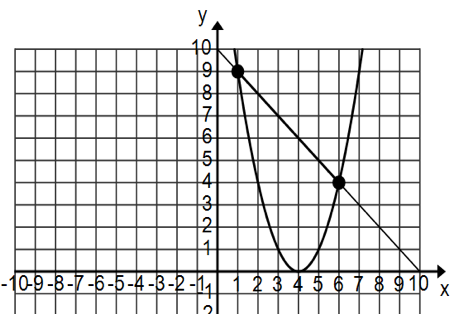### A circus clown walks down a straight rope from a point 9 feet above the ground as shown. At the same time, a trapeze artist swings down a parabolic path shown. After crossing the trapeze artist for the first time at 9 feet, approximately how far does the clown walk down the rope before meeting the trapeze artist again?

 A $3$ B $5$ C $6$ D $7$
Question 16 Explanation:

The first point where they meet is $(1, 9)$. The second point of meeting is $(6, 4)$. Use the distance formula to find the distance that the clown walked down the rope:

$d$ $= \sqrt{(y_2 − y_1)^2 + (x_2 − x_1)^2}$

$= \sqrt{(4 − 9)^2 + (6 − 1)^2}$

$= \sqrt{25 + 25} = 7.07 \text{ feet}$

This is approximately equal to 7 feet. Note that the question asks “approximately how far”, thereby giving you a clue to round your answer.
 Question 17

### Use the following information for questions 17 and 18.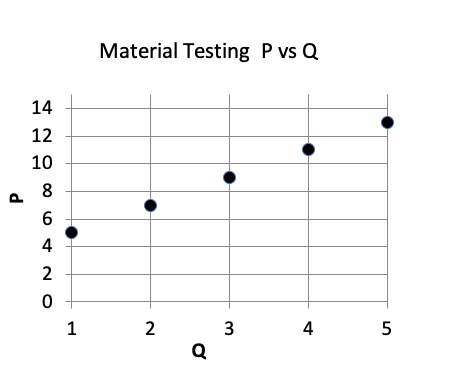### A materials engineer is testing three properties $P$, $Q$, and $R$ of a new alloy. All three of them have the same units of measurement. Scatterplots showing the relation between $P$ and $Q$ and the relation between $Q$ and $R$ are shown above. Which of the following models best describes the relation between $P$ and $R$ ?

 A $P = 7 − 2R$ B $P = 2R − 7$ C $P = R + 5$ D $P = R − 5$
Question 17 Explanation:
The correct answer is (B). First, find the relationship between $P$ and $Q$ by listing the scatterplot values for $P$ and $Q$.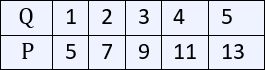The relation can be expressed as $P = 2Q + 3$, where $2$ is the slope and $3$ is the $y$-intercept of the line connecting the points.

Now find the relation between $Q$ and $R$.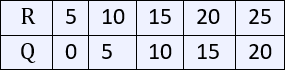The relation between $Q$ and $R$ is $Q = R − 5$, where $1$ (not shown) is the slope and $−5$ is the $y$-intercept of the line connecting the points.

The question asks for the relationship between $P$ and $R$. You have $P = 2Q + 3$ and $Q = R − 5$.

Substituting $“R − 5”$ for $Q$ in the first equation gives:

$P = 2(R − 5) + 3$

$= 2R − 10 + 3$

$= 2R − 7$
 Question 18### If the property $Q = 6$ units, then what is the ratio of $R$ to $P$ ?

 A $\dfrac{7}{15}$ B $\dfrac{11}{15}$ C $\dfrac{13}{15}$ D $\dfrac{17}{15}$
Question 18 Explanation:
The correct answer is (B). As shown in the scatterplots:

When $Q = 6$, $R = Q + 5$
$R = 6 + 5$
$R = 11$

and

$P = 2Q + 3$
$P = 2 \cdot 6 + 3$
$P = 15$

The ratio of $R$ to $P = \dfrac{11}{15}$
 Question 19

### The ratio of poor people to rich people in a town in the year 1990 was 6 to 1. The same ratio in the year 2000 was 10 to 3. Assuming that the population of the town remained the same, what would be the ratio of rich people in the town in 2000 to rich people in the town in 1990?

 A $\dfrac{13}{7}$ B $\dfrac{3}{1}$ C $\dfrac{21}{13}$ D $\dfrac{9}{5}$
Question 19 Explanation:

In 1990, $\frac{\text{poor}}{\text{rich}} = \frac{6}{1}$

For every 7 people in town 1 is rich and 6 are poor. Thus, $\frac{1}{7}$ of the town is rich.

In 2000, $\frac{\text{poor}}{\text{rich}} = \frac{10}{3}$

For every 13 people in town 3 are rich and 10 are poor. Thus, $\frac{3}{13}$ of the town is rich.

Since the population of the town did not change, we can find a common denominator for $\frac{1}{7}$ and $\frac{3}{13}$ and compare the proportion of rich people:

$\dfrac{1}{7} = \dfrac{1(13)}{7(13)} = \dfrac{13}{91} \;$ and $\; \dfrac{3}{13} = \dfrac{3(7)}{13(7)} = \dfrac{21}{91}$

Therefore, the ratio of rich people in town in 2000 vs 1990:

$\dfrac{21}{13}$

You can also find the answer by taking $\frac{3}{13} / \frac{1}{7} = \frac{21}{13}$
 Question 20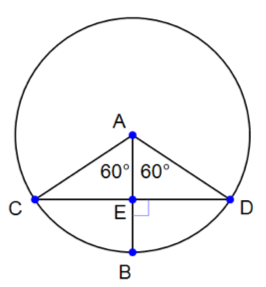### Based on the diagram above, if chord $CD$ has a length of 10, what is the length of $AB$ ?

 A $10$ B $\dfrac{10\sqrt{3}}{3}$ C $5\sqrt{3}$ D $\dfrac{10\sqrt{2}}{3}$
Question 20 Explanation:
The correct answer is (B). You can use the 30–60–90 triangles created when triangle $ACD$ is divided into two. This gives:

$CE = 5$, $AE = \dfrac{5\sqrt{3}}{3}$, and $AC = \dfrac{10\sqrt{3}}{3}$

$AB$ is a radius of the circle and has the same value as $AC$.
 Question 21

### If a circle with its origin at $(0, 0)$ passes through the point $(–4, 0)$, then which one of the following points is on the circumference of the circle?

 A $(–4, 1)$ B $(0, 5)$ C $(–1, \sqrt{15})$ D $(2, \sqrt{14})$
Question 21 Explanation:
The correct answer is (C). Since the circle has its origin at $(0, 0)$ and passes through $(–4, 0)$, its radius has a length of $4$ units. So, any other point on the circle must satisfy the equation:

$x^2 + y^2 = r^2 = 4^2 = 16$

Of the answer choices given, only $(–1, \sqrt{15})$ satisfies the equation:

$(–1)^2 + (\sqrt{15})^2$

$= 1 + 15 = 16$
 Question 22

### A stone is thrown from the ground along a parabolic path defined by the function $y = −x^2 + 4x$, where $x$ and $y$ are in feet. The stone hits the tip of a vertical pole that is $4$ feet tall at the vertex of its path. How far is the pole from the point where the stone was thrown?

 A $1$ B $2$ C $4$ D $16$
Question 22 Explanation:
The correct answer is (B). The stone takes a parabolic path which opens downward, since the coefficient of $x^2$ is $−1$. The path of the stone intersects the tip of the pole that is $4$ feet tall. This can be represented as $y = 4$. Together, you have a linear quadratic system.

$y = −x^2 + 4x$

$y = 4$

Equate them and solve for $x$.

$−x^2 + 4x = 4$

$x^2 − 4x = –4$

$x^2 − 4x + 4 = 0$

$x^2 − 2x − 2x + 4 = 0$

$x(x − 2) − 2(x − 2) = 0$

$(x − 2)(x − 2) = 0$

$(x − 2) = 0$

$x = 2$, $y = 4$

The stone touches the pole at $x = 2$.
 Question 23

### In a housing community, 15 people play Tennis, 20 people play Soccer, and 25 people play Baseball. There are 5 people who play Tennis and Soccer but not Baseball. There are 4 people who play Tennis and Baseball but not Soccer. There are 6 people who play Soccer and Baseball but not Tennis. There are 35 people in the housing community. How many people play all three games?

 A $3$ B $4$ C $5$ D $9$
Question 23 Explanation:
The correct answer is (C). Place the numbers from the problem in the Venn diagram:Let $x$ be the number of people who play all the games.

Those who play tennis only is:
$15 − 5 − 4 – x = 6 − x$

Those who play soccer only is:
$20 − 5 − 6 − x = 9 − x$

Those who play baseball only is:
$25 − 4 − x − 6 = 15 − x$

There are a total of 35 people, so each section of the Venn diagram must sum to 35. Setup an equation:

$(6 − x) + 5 + x + 4$ $+ (9 − x) + 6 + (15 − x)$ $= 35$

$45 − 2x = 35$

$2x = 10$

$x = 5$. So, $5$ people play all the games.
 Question 24

### When an object is in motion, its velocity $v$ is related to its kinetic energy $E$, and its mass $m$, by the formula $v = \sqrt{\frac{2E}{m}}$. If the velocity of an object of mass $m_2$ is twice the velocity of an object of mass $m_1$, and the kinetic energy of both objects are the same, then what is the ratio of $\dfrac{m_1}{m_2}$?

 A $1$ B $2$ C $4$ D $8$
Question 24 Explanation:
The correct answer is (C). Though strange looking, this problem is easy to solve. There are 2 objects, of mass $m_1$ and $m_2$.

Let $v_1$ and $v_2$ be their velocities. Their kinetic energies are the same.

$v_1 = \sqrt{\dfrac{2E}{m_1}} \;$ and $\; v_2 = \sqrt{\dfrac{2E}{m_2}}$

$v_2 = 2v_1$ (given fact)

So, $\sqrt{\dfrac{2E}{m_2}} = 2 \sqrt{\dfrac{2E}{m_1}}$

Square both sides (Don’t forget to square the 2):

$\dfrac{2E}{m_2} = 4 \dfrac{2E}{m_1}$

$\dfrac{2E}{m_2} = \dfrac{8E}{m_1}$

Cross multiply to get:

$(m_2)(8E) = (2E)(m_1)$

$\require{cancel} \dfrac{(m_2)(8\cancel{E})}{2\cancel{E}} = \dfrac{(\cancel{2E})(m_1)}{\cancel{2E}}$

$\dfrac{8 m_2}{2} = m_1$

$4m_2 = m_1$

Therefore, $\dfrac{m_1}{m_2} = 4$
 Question 25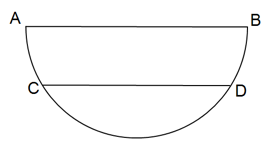### In the figure above, a semicircle of radius $r$ has a chord $\overline{\text{CD}}$ that is parallel to diameter $\overline{\text{AB}}$ and is $\frac{1}{4}$ of $\overline{\text{AB}}$. What is the distance between chords $\overline{\text{AB}}$ and $\overline{\text{CD}}$ in terms of $r$ ?

 A $\sqrt{15}r$ B $\dfrac{\sqrt{15}}{4}r$ C $\dfrac{\sqrt{15}}{2}r$ D $\sqrt{4}r$
Question 25 Explanation:Let the center of the semicircle be the point $O$. Draw a line $OC$ and $OP$. So, $OA = r OC = r$.

$OP$ is the distance between the chords. $OPC$ is a right-angled triangle: $OC^2 = OP^2 + PC^2$

$r^2 = OP^2 + \left( \dfrac{r}{4}\right)^2$

$OP^2 = r^2 − \dfrac{r^2}{16} = \dfrac{15}{16} r^2$

$OP = \dfrac{\sqrt{15}}{4}r$
 Question 26

### If $\cos x = −p$ where the radian measure of angle $x$ is such that $π < x < \frac{3π}{2}$ and $\cos y = p$, then $y$ can be which one of the following?

 A $π − x$ B $x − \dfrac{π}{2}$ C $x + 2π$ D $x − 2π$
Question 26 Explanation:

Find an angle, $x$, that satisfies the requirement $π < x < \frac{3π}{2}$. $x = \frac{5π}{4}$ is a logical choice.

It is given that $\cos{x} = −p$. So, $p = −\cos{x}$.

$p = −\cos\left(\dfrac{5π}{4}\right)$

Using our calculator (in radians) we find: $−\cos\left(\dfrac{5π}{4}\right) = \dfrac{\sqrt{2}}{2}$

$p = \dfrac{\sqrt{2}}{2}$

It is also given that $\cos{y} = p$. So, $\cos{y} = \dfrac{\sqrt{2}}{2}$.

Try each multiple choice answer to find the one that works.

If $y = π − x$ (Answer choice "A") we have:

$\cos\left(π − \dfrac{5π}{4}\right)$

Plugging this into our calculator we find:

$\cos\left(π − \dfrac{5π}{4}\right) = \dfrac{\sqrt{2}}{2}$

This solution works — we have our answer. Below is an alternative way to solve this: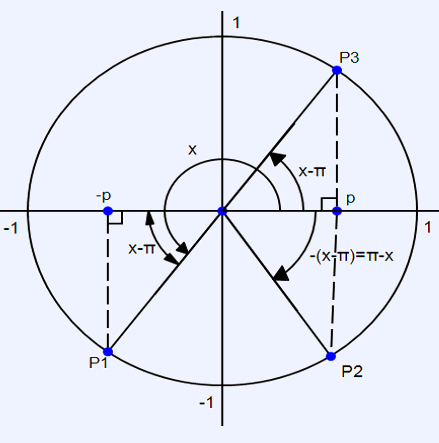Note that the angles are in radians. The angle $x$ is such that $π < x < \frac{3π}{2}$. So, the angle is in the third quadrant. It is given that $\cos x = −p$. Mark a point P1 at an angle of $x$ radians. Note that the reference angle of point P1 is $x − π$.

Since $\cos y = p$, and cosine is positive in 1st and 4th quadrants, the point in the unit circle for which $\cos y = p$ is in the 1st quadrant (P3) or in the 4th quadrant (P2). If it is in the 1st quadrant, the angle of the point P3 is $x − π$. This could be the answer but is not in the answer choices. If the point is in the 4th quadrant (P2), then its reference angle is $(x − π)$, but since the angle is measured counterclockwise, the angle of the point P2 is $−(x − π) = π − x$.

So, $\cos (π − x) = p$. Since it is given that $\cos y = p$, it follows that $y = π − x$.
 Question 27

### The product of $(5 + 5i) \cdot (5 − 6i)$ can be expressed in the form $a + bi$. What is the sum $a + b$ ?

 A $\text{Click}$ $\text{here}$ $\text{to}$ $\text{see}$ $\text{the}$ $\text{correct}$ $\text{answer.}$
Question 27 Explanation:
The correct answer is $50$.

Calculators such as the TI-36X Pro and the CASIO fx-991EX, both permitted for use on the test, make this question very easy. Both are capable of finding the product of complex numbers.

Simply typing in $(5 + 5i)(5 − 6i)$ yields $55 − 5i$.

$a = 55$ and $b = −5$, so $a + b = 55 + −5 = 50$.

Here is an alternative way to solve this:

$(5 + 5i) \cdot (5 − 6i)$

$= (5)(5) + (5)(− 6i)$ $+ \, (5i)(5) + (5i)(− 6i)$

$= 25 − 30i + 25i − 30i^2$

$= 25 − 30i + 25i − 30(−1)$

$= 25 − 30i + 25i + 30$

$= 55 − 5i$

The sum of $a$ and $b = 55 + (−5) = 50$
 Question 28### A cylindrical hole with a radius, $r$, of $5$ cm is cut through a regular hexagonal prism with a height, $h$, of $10$ cm and a side length, $s$, of $10$ cm. If the density of this object is $3.2$ grams/cm$^3$, what is the mass of the hexagonal prism after the hole has been made? (figure not drawn to scale) Volume of regular hexagonal prism $= \left(\dfrac{3\sqrt{3}}{2}\right)(s^2)(h)$ Volume of cylinder $= (π)(r^2)(h)$

 A $\text{Click}$ $\text{here}$ $\text{to}$ $\text{see}$ $\text{the}$ $\text{correct}$ $\text{answer.}$
Question 28 Explanation:
The correct answer is $5801$.

The volume of the cylinder is:

$(π)(5^2)(10) = 250π$ cm$^3$

The volume of the rectangular hexagonal prism is:

$\left(\dfrac{3\sqrt{3}}{2}\right)(10^2)(10) = 1500\sqrt{3}$ cm$^3$

The volume of the prism after the hole has been made:

$1500\sqrt{3} − 250π ≅ 1812.68$ cm$^3$

The density is: $3.2$ g/cm$^3$

$\text{Mass} = \text{Volume} \cdot \text{Density}$

The mass of the prism after the hole has been made:

$1812.68 \cdot 3.2 = 5800.58$ g

Rounded to the nearest whole gram:

$5801$ g
 Question 29

The New Express Bank issues its credit cards worldwide. When a customer makes a purchase using these credit cards in a foreign currency, the bank converts the purchase price at the daily foreign rate and then charges a 5% fee on the converted cost.

### Marla lives in the USA, and is currently on vacation in Shanghai, China. For a purchase that she made in Shanghai for 75 Yuans, the bank posted a charge of $12.42 to her account, including the 5% fee. What Foreign Exchange Rate in (Chinese Yuan per US dollar) was used to calculate Marla’s purchase? Round your answer to the nearest hundredths. ### (Write down your answer.)  A$\text{Click}\text{here}\text{to}\text{see}\text{the}\text{correct}\text{answer.}$Question 29 Explanation: The correct answer is$6.34$. Solve as shown below:$\text{Dollars} + \text{Fee} = \$12.42$

$\text{Dollars} + 5\% (\text{Dollars})$ $= 12.42$

$x + 0.05x = 12.42$

$1.05x = 12.42$

$x = \dfrac{12.42}{1.05}$

$x = 11.8286$

$\dfrac{75~¥}{\$11.8286} = \dfrac{x~¥}{\$1}$

$(11.8286)(x) = (75)(1)$

$x= 6.34 \text{ Dollars}$
Once you are finished, click the button below. Any items you have not completed will be marked incorrect.
There are 29 questions to complete.
 ← List →